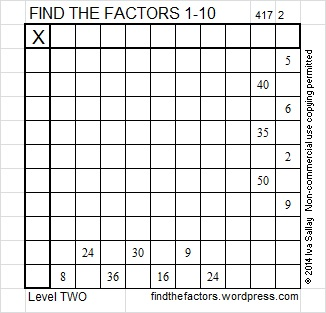# 103 and Level 2

• 103 is a prime number.
• Prime factorization: 103 is prime.
• The exponent of prime number 103 is 1. Adding 1 to that exponent we get (1 + 1) = 2. Therefore 103 has exactly 2 factors.
• Factors of 103: 1, 103
• Factor pairs: 103 = 1 x 103
• 103 has no square factors that allow its square root to be simplified. √103 ≈ 10.14889How do we know that 103 is a prime number? If 103 were not a prime number, then it would be divisible by at least one prime number less than or equal to √103 ≈ 10.1 Since 103 cannot be divided evenly by 2, 3, 5, or 7, we know that 103 is a prime number.

103 is never a clue in the FIND THE FACTORS puzzles.This week’s puzzles and last week’s factors: 10 Factors 2014-04-28## 2 thoughts on “103 and Level 2”

1.Steve Morris

I like your explanation of how to quickly show that 103 is a prime number. I didn’t know that.

2.ivasallay

Thank you. It is a powerful time saver.

This site uses Akismet to reduce spam. Learn how your comment data is processed.